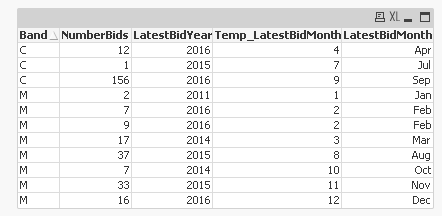# QlikView App Dev

Discussion Board for collaboration related to QlikView App Development.

Announcements
Live chat with experts, bring your API Integration questions. June 15th, 10 AM ET. REGISTER TODAY
cancel
Showing results for
Search instead for
Did you mean:Not applicable

## Converting DOB to age and then banding it

Hi,

We have the following expression which converts DOB (dd/mm/yyyy) into Age (e.g 28):

year(Today()) - year(DOB) as Age,

We then want to band these ages. For banding we use an expression which always works:

IF ([Age]>=16 and [Age] <=24,'16 to 24',
IF ([Age]>=25 and [Age] <=34,'25 to 34',
IF ([Age]>=35 and [Age] <=44,'35 to 44',
IF ([Age]>=45 and [Age] <=54,'45 to 54',
IF ([Age]>=55 and [Age] <=64,'55 to 64',
IF ([Age]>=65 and [Age] <=74,'65 to 74',
IF ([Age]>=75,'75+'))))))) as AgeBand

So combined it is as follows:

year(Today()) - year(DOB) as Age,
IF ([Age]>=16 and [Age] <=24,'16 to 24',
IF ([Age]>=25 and [Age] <=34,'25 to 34',
IF ([Age]>=35 and [Age] <=44,'35 to 44',
IF ([Age]>=45 and [Age] <=54,'45 to 54',
IF ([Age]>=55 and [Age] <=64,'55 to 64',
IF ([Age]>=65 and [Age] <=74,'65 to 74',
IF ([Age]>=75,'75+'))))))) as AgeBand

However, it doesn't like it in the script when we combine. Can someone help?

Attached is the raw data if it helps.

Chris

1 Solution

Accepted SolutionsMVP

Try this in that case:

Table:

LOAD Band,

NumberBids,

LatestBidYear,

LatestBidMonth,

DOB,

Year(Today()) - Year(DOB) as Age,

IF (Year(Today()) - Year(DOB)>=16 and Year(Today()) - Year(DOB) <=24,'16 to 24',

IF (Year(Today()) - Year(DOB)>=25 and Year(Today()) - Year(DOB) <=34,'25 to 34',

IF (Year(Today()) - Year(DOB)>=35 and Year(Today()) - Year(DOB) <=44,'35 to 44',

IF (Year(Today()) - Year(DOB)>=45 and Year(Today()) - Year(DOB) <=54,'45 to 54',

IF (Year(Today()) - Year(DOB)>=55 and Year(Today()) - Year(DOB) <=64,'55 to 64',

IF (Year(Today()) - Year(DOB)>=65 and Year(Today()) - Year(DOB) <=74,'65 to 74',

IF (Year(Today()) - Year(DOB)>=75,'75+'))))))) as AgeBand

FROM

[calenderqv (2).xlsx]

(ooxml, embedded labels, table is Sheet1);

7 RepliesMVP

I think you need to do the banding in the preceding load. Are you doing it in the same load for some reason?MVP

Like this?Table:

LOAD *,

IF ([Age]>=16 and [Age] <=24,'16 to 24',

IF ([Age]>=25 and [Age] <=34,'25 to 34',

IF ([Age]>=35 and [Age] <=44,'35 to 44',

IF ([Age]>=45 and [Age] <=54,'45 to 54',

IF ([Age]>=55 and [Age] <=64,'55 to 64',

IF ([Age]>=65 and [Age] <=74,'65 to 74',

IF ([Age]>=75,'75+'))))))) as AgeBand;

LOAD Band,

NumberBids,

LatestBidYear,

LatestBidMonth,

DOB,

Year(Today()) - Year(DOB) as Age

FROM

[calenderqv (2).xlsx]

(ooxml, embedded labels, table is Sheet1);Not applicable
Author

Needs to be in the same load as we want to avoid creating unnecessary steps.

We will be receiving a large spreadsheet and then want to convert into Qlikview quickly on one script if we can.

ChrisMVP

Try this in that case:

Table:

LOAD Band,

NumberBids,

LatestBidYear,

LatestBidMonth,

DOB,

Year(Today()) - Year(DOB) as Age,

IF (Year(Today()) - Year(DOB)>=16 and Year(Today()) - Year(DOB) <=24,'16 to 24',

IF (Year(Today()) - Year(DOB)>=25 and Year(Today()) - Year(DOB) <=34,'25 to 34',

IF (Year(Today()) - Year(DOB)>=35 and Year(Today()) - Year(DOB) <=44,'35 to 44',

IF (Year(Today()) - Year(DOB)>=45 and Year(Today()) - Year(DOB) <=54,'45 to 54',

IF (Year(Today()) - Year(DOB)>=55 and Year(Today()) - Year(DOB) <=64,'55 to 64',

IF (Year(Today()) - Year(DOB)>=65 and Year(Today()) - Year(DOB) <=74,'65 to 74',

IF (Year(Today()) - Year(DOB)>=75,'75+'))))))) as AgeBand

FROM

[calenderqv (2).xlsx]

(ooxml, embedded labels, table is Sheet1);MVP

I personally would not use

Year(Today())-Year(DOB) as Age

if you want to consider Age as 'completed years'.

There is Age() function in QV that returns this value as it its commonly used for age calculations.

Age(Today(1), DOB) as AgeEmployee

You can use age() function, it would be more conciseMVP

Clever -

I agree with you and Stefan, but the reason I did not propose that was because it seems that Chris have a specific way to calculate age and I did not want to change it. If by date is what he wants, then yes Age() function is the way to goBest,

Sunny